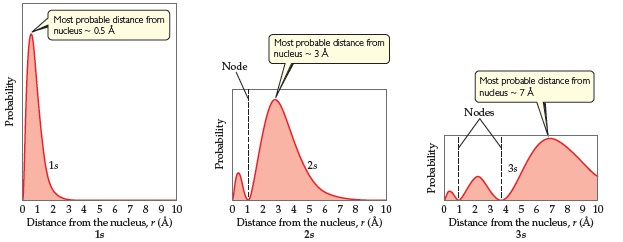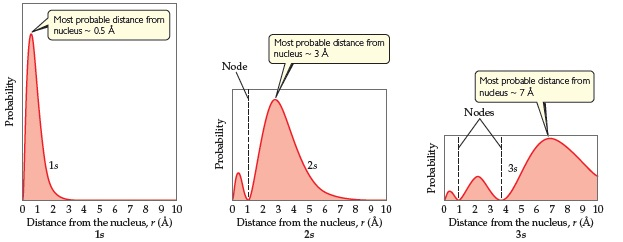# Problem: With reference to the figure below, what is the relationship between the number of nodes in an s orbital and the value of the principal quantum number?Radial probability functions for the 1s, 2s, and 3s orbitals of hydrogen. These plots show the probability of finding the electron as a function of distance from the nucleus. As  n  increases, the most likely distance at which to find the electron (the highest peak) moves farther from the nucleus.

###### FREE Expert Solution

We’re being asked to determine the relationship between the number of nodes in an s orbital and the value of the principal quantum number.

Let's refer to the given figure below:For 1s:

80% (393 ratings)###### Problem Details

With reference to the figure below, what is the relationship between the number of nodes in an s orbital and the value of the principal quantum number?Radial probability functions for the 1s, 2s, and 3s orbitals of hydrogen. These plots show the probability of finding the electron as a function of distance from the nucleus. As  n  increases, the most likely distance at which to find the electron (the highest peak) moves farther from the nucleus.

Frequently Asked Questions

What scientific concept do you need to know in order to solve this problem?

Our tutors have indicated that to solve this problem you will need to apply the Quantum Numbers: Nodes concept. You can view video lessons to learn Quantum Numbers: Nodes. Or if you need more Quantum Numbers: Nodes practice, you can also practice Quantum Numbers: Nodes practice problems.

What professor is this problem relevant for?

Based on our data, we think this problem is relevant for Professor Stoltzfus' class at OSU.